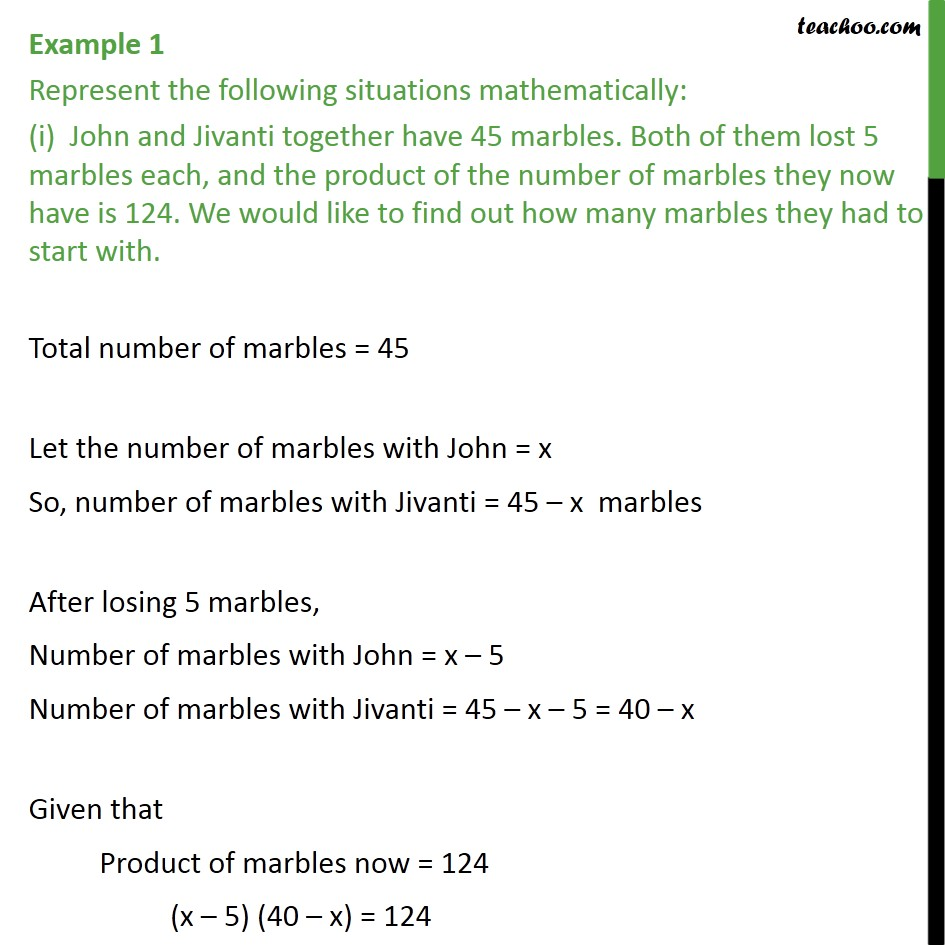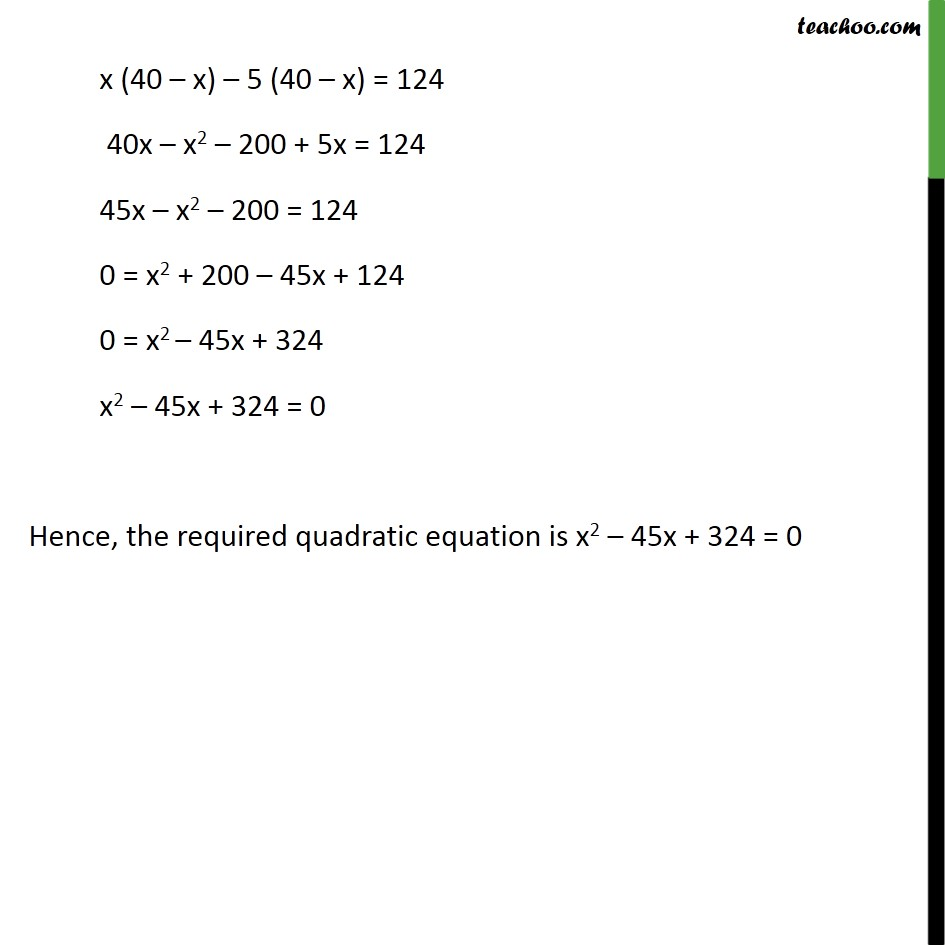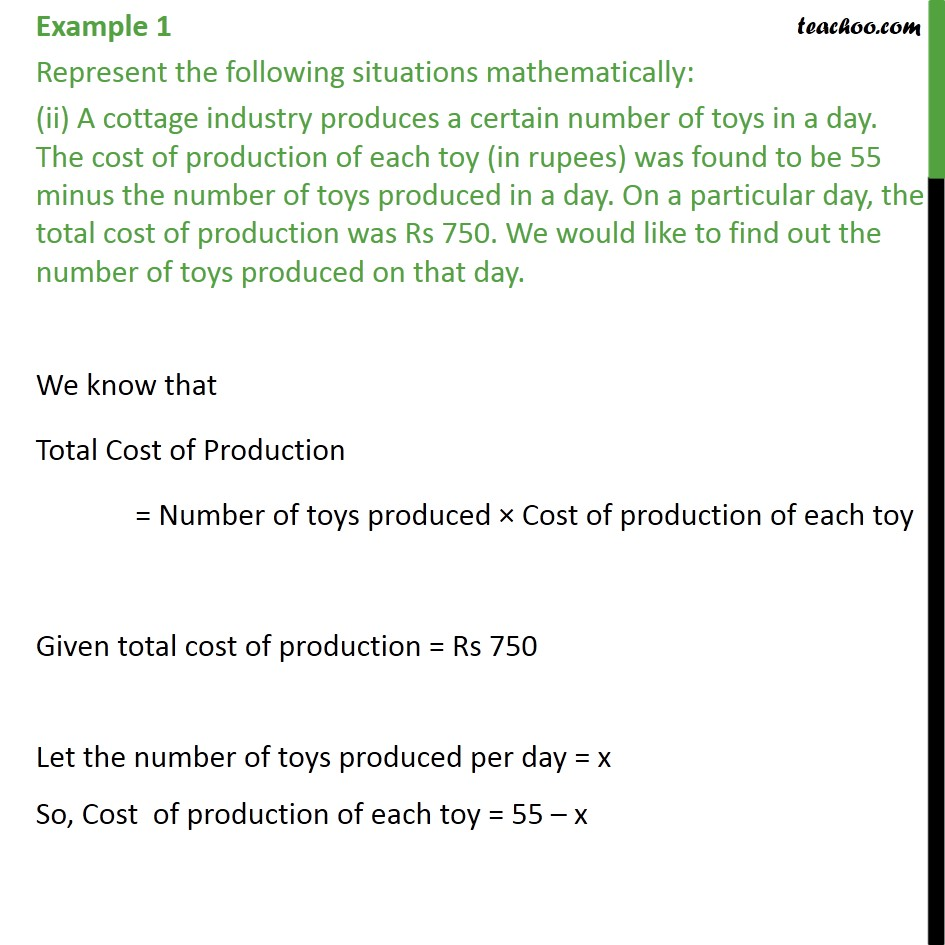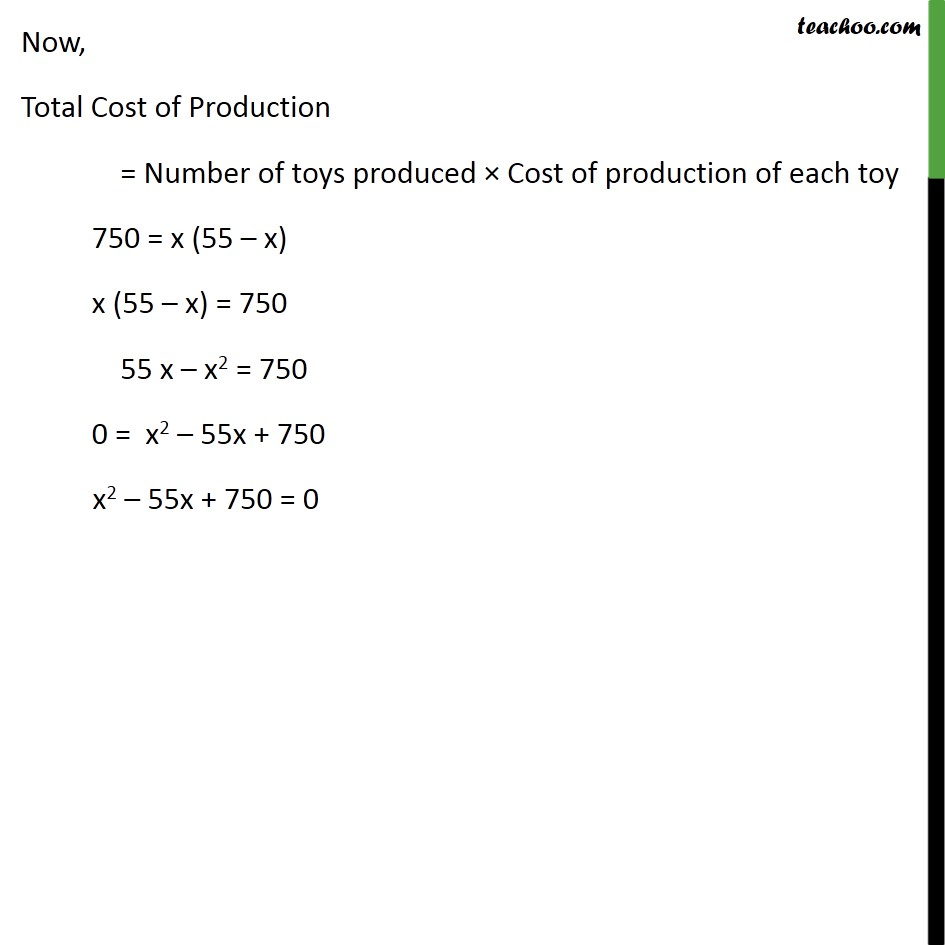Learn all Concepts of Chapter 4 Class 10 (with VIDEOS). Check - Quadratic Equations - Class 101. Chapter 4 Class 10 Quadratic Equations
2. Serial order wise
3. Examples

Transcript

Example 1 Represent the following situations mathematically: (i) John and Jivanti together have 45 marbles. Both of them lost 5 marbles each, and the product of the number of marbles they now have is 124. We would like to find out how many marbles they had to start with. Total number of marbles = 45 Let the number of marbles with John = x So, number of marbles with Jivanti = 45 – x marbles After losing 5 marbles, Number of marbles with John = x – 5 Number of marbles with Jivanti = 45 – x – 5 = 40 – x Given that Product of marbles now = 124 (x – 5) (40 – x) = 124 x (40 – x) – 5 (40 – x) = 124 40x – x2 – 200 + 5x = 124 45x – x2 – 200 = 124 0 = x2 + 200 – 45x + 124 0 = x2 – 45x + 324 x2 – 45x + 324 = 0 Hence, the required quadratic equation is x2 – 45x + 324 = 0 Example 1 Represent the following situations mathematically: (ii) A cottage industry produces a certain number of toys in a day. The cost of production of each toy (in rupees) was found to be 55 minus the number of toys produced in a day. On a particular day, the total cost of production was Rs 750. We would like to find out the number of toys produced on that day. We know that Total Cost of Production = Number of toys produced × Cost of production of each toy Given total cost of production = Rs 750 Let the number of toys produced per day = x So, Cost of production of each toy = 55 – x Now, Total Cost of Production = Number of toys produced × Cost of production of each toy 750 = x (55 – x) x (55 – x) = 750 55 x – x2 = 750 0 = x2 – 55x + 750 x2 – 55x + 750 = 0

Examples